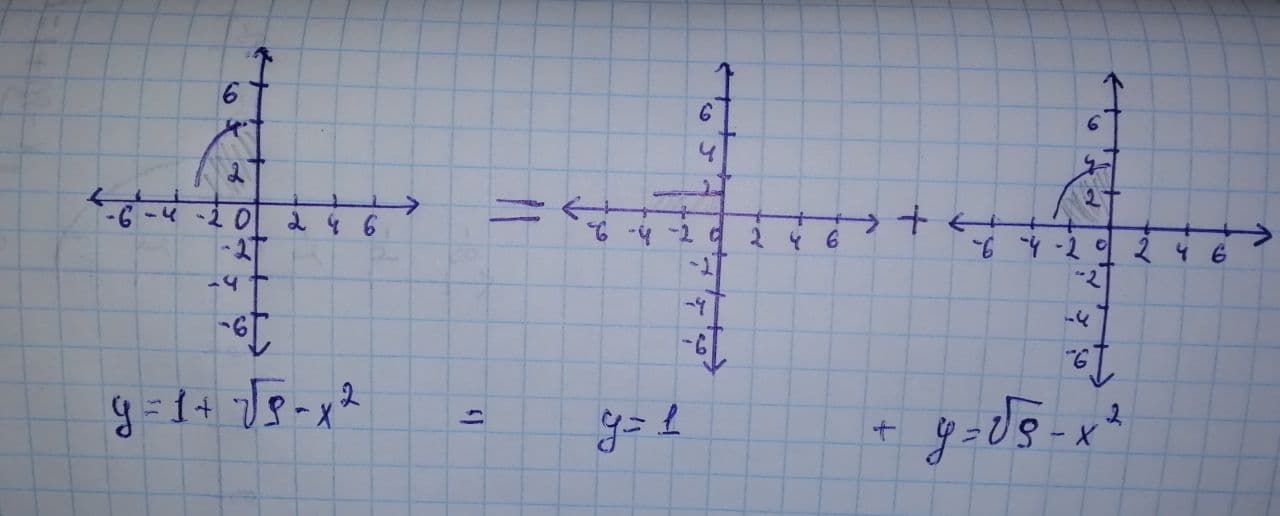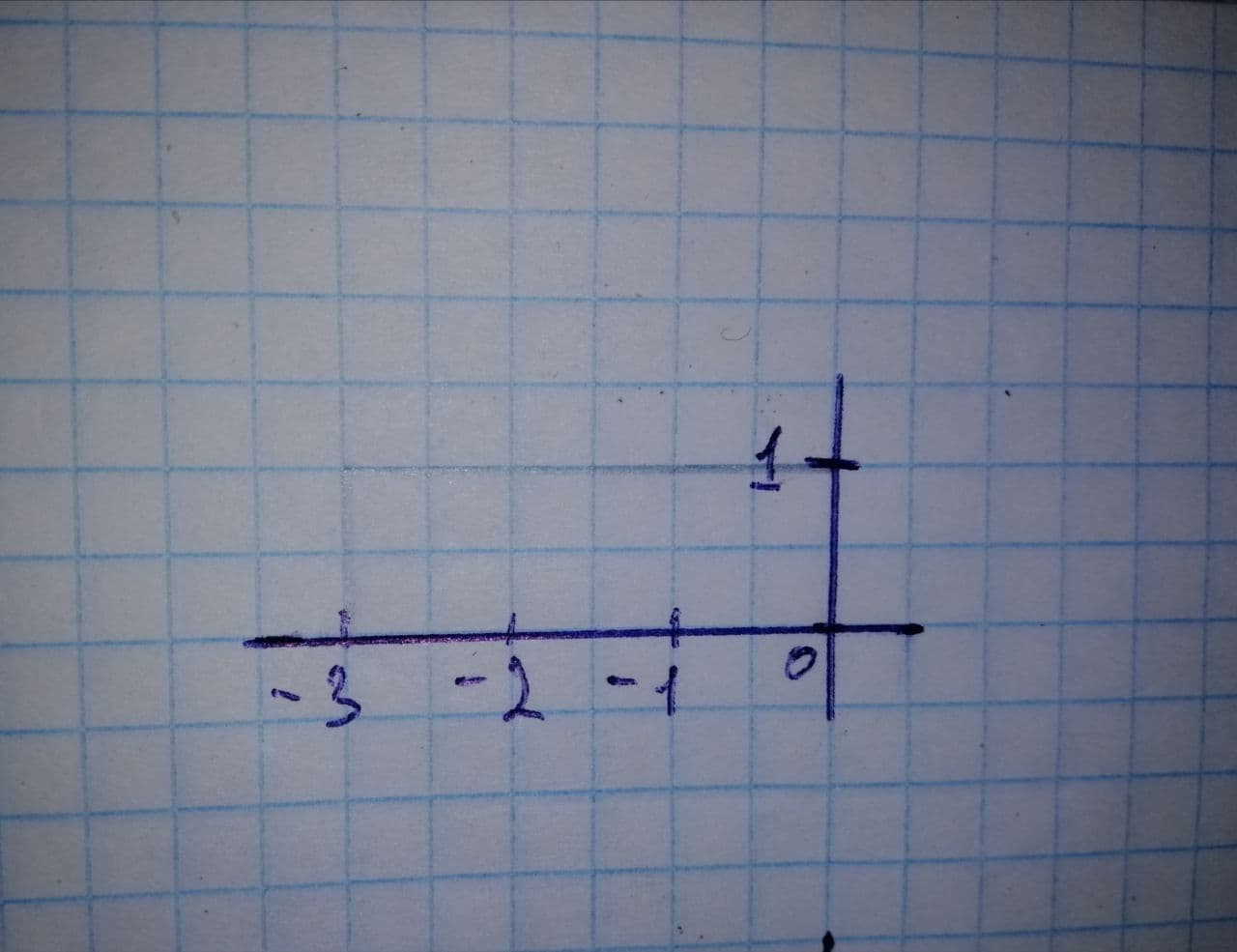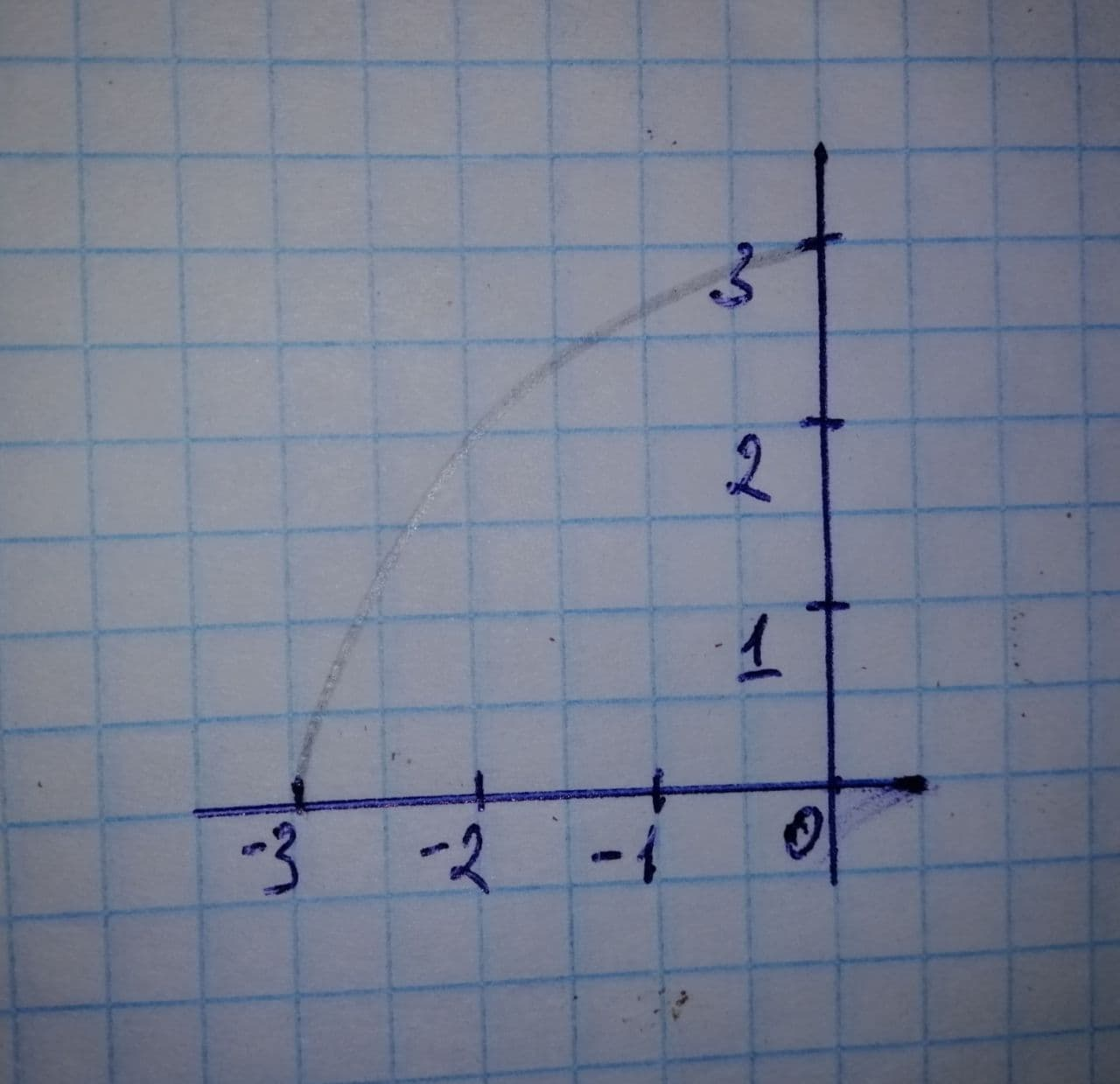Mary Buchanan

2021-12-19

Evaluate the integral by interpreting it in terms of areas. integral:
${\int }_{-3}^{0}\left(1+\sqrt{9-{x}^{2}}\right)dx$lenkiklisg7

Expert

Step 1
First we break the integral into two parts:
${\int }_{-3}^{0}\left(1+\sqrt{9-{x}^{2}}\right)dx={\int }_{-3}^{0}1dx+{\int }_{-3}^{0}\sqrt{9-{x}^{2}}dx$
The first term ${\int }_{-3}^{0}1dx$ represents area of rectangle with base $b=3$ and height $h=1$
${\int }_{-3}^{0}1dx=b×h=3$
${\int }_{-3}^{0}\sqrt{9-{x}^{2}}dx$ represent area of quarter circle with radius $r=3$
${\int }_{-3}^{0}\sqrt{9-{x}^{2}}dx=\frac{1}{4}\pi ×{3}^{2}=\frac{9\pi }{4}$
${\int }_{-3}^{0}\left(1+\sqrt{9-{x}^{2}}\right)dx={\int }_{-3}^{0}1dx+{\int }_{-3}^{0}\sqrt{9-{x}^{2}}dx$
${\int }_{-3}^{0}\left(1+\sqrt{9-{x}^{2}}\right)dx=3+\frac{9\pi }{4}$Lakisha Archer

Expert

Step 1
The given integral is
$I={\int }_{-3}^{0}\left(1+\sqrt{9-{x}^{x}}\right)dx$
$I={\int }_{-3}^{0}1×x+{\int }_{-3}^{0}\sqrt{9-{x}^{2}}dx$
$I={A}_{1}+{A}_{2}$
we can plot area A1 as followswe can plot area A2 as followsHence we can calculate the integral as
$I=W×H+\frac{\pi {r}^{2}}{4}$
$I=3×1+\frac{\pi {\left(3\right)}^{2}}{4}$
$I=3+\frac{9\pi }{4}$nick1337

Expert

The expression you are having trouble with seems to be incorrect.
You have
$f\left(x\right)=1+\sqrt{9-{x}^{2}}$
Since
$a=-3$ and $b=0$
you have

$f\left({x}_{k}\right)=1+{\sqrt{9-\left(-3+\frac{3k}{n}\right)}}^{2}$
$=1+\sqrt{18}\frac{k}{n}-9\frac{{k}^{2}}{{n}^{2}}$
$=1+\frac{3}{n}\sqrt{2kn-{k}^{2}}$
From this, we see that
$f\left({x}_{k}\right)\mathrm{\Delta }x=\frac{3}{n}\left(1+\frac{3}{n}\sqrt{2kn-{k}^{2}}\right)$
$=\frac{3}{n}+\frac{9}{{n}^{2}}\sqrt{2kn-{k}^{2}}$
Then the final expression should be
$\underset{n⇒\mathrm{\infty }}{lim}\left[\sum _{k=1}^{n}\left(\frac{3}{n}+\frac{9}{{n}^{2}}\sqrt{2kn-{k}^{2}}\right)\right]$

Do you have a similar question?